# Python – How to make a terminal progress bar using tqdm

## Python | How to make a terminal progress bar using tqdm

Whether you’re installing software, loading a page or doing a transaction, it always eases your mind whenever you see that small progress bar giving you an estimation of how long the process would take to complete or render. If you have a simple progress bar in your script or code, it looks very pleasing to the eye and gives proper feedback to the user whenever he executes the code. You can use the Python external library tqdm, to create simple & hassle-free progress bars which you can add in your code and make it look lively!

## Installation

Open your command prompt or terminal and type:

`pip install tqdm`

If you are using Python3 then type:

`pip3 install tqdm`

This command would successfully install the library on your computer and is now ready to use.

## Usage

Using `tqdm` is very simple, you just need to add your code between `tqdm()` after importing the library in your code. You need to make sure that the code you put in between the tqdm() function must be iterable or it would not work at all.

Let us see the following example that would help you understand better:

Example:

 `from` `tqdm ``import` `tqdm` ` ` ` ` `for` `i ``in` `tqdm(``range``(``int``(``9e6``))):` `    ``pass`

Output: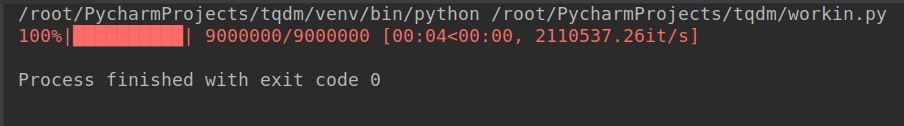Now that we know how to implement tqdm, let’s take a look at some of the important parameters it offers and how it can be used to tweak the progress bar.

• desc: You can use this parameter to specify the description of your progress bar as follows:Syntax:
`tqdm (self, iterable, desc= “Text You want”)`

Example:

 `from` `tqdm ``import` `tqdm` `from` `time ``import` `sleep` ` ` ` ` `for` `i ``in` `tqdm(``range``(``0``, ``100``), desc ``=``"Text You Want"``):` `    ``sleep(.``1``)`

Output: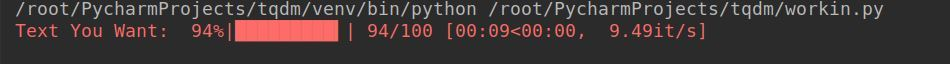• total: This is used to specify the total number of expected iterations if not specified already or needs modification.Syntax:
`tqdm (self, iterable, total= 500)`

Example:

 `from` `tqdm ``import` `tqdm` `from` `time ``import` `sleep` ` ` ` ` `for` `i ``in` `tqdm(``range``(``0``, ``100``), total ``=` `500``,` `              ``desc ``=``"Text You Want"``):` `    ``sleep(.``1``)`

Output: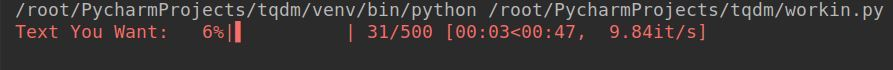• disable: This parameter can be used if you want to completely disable the progress bar.Syntax:
`tqdm (self, iterable, disable=True)`

Example:

 `from` `tqdm ``import` `tqdm` `from` `time ``import` `sleep` ` ` ` ` `for` `i ``in` `tqdm(``range``(``0``, ``100``), disable ``=` `True``,` `               ``desc ``=``"Text You Want"``):` `    ``sleep(.``1``)` ` ` `print``(``"Iteration Successful"``)`

Output: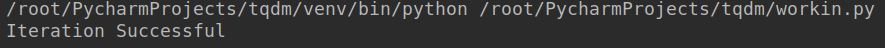• ncols: This parameter is used to specify the entire width of the output message. If left unspecified it remains dynamic to the size of the window. This can be fixed through the `ncols` parameter.Syntax:
`tqdm (self, iterable, ncols= 100)`

Example:

 `from` `tqdm ``import` `tqdm` `from` `time ``import` `sleep` ` ` ` ` `for` `i ``in` `tqdm(``range``(``0``, ``100``), ncols ``=` `100``,` `               ``desc ``=``"Text You Want"``):` `    ``sleep(.``1``)`

Output: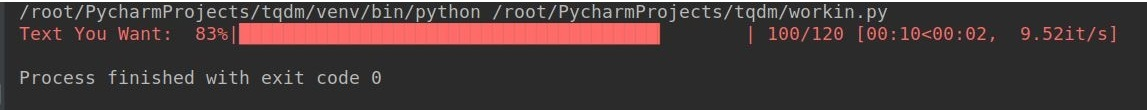• mininterval: You can easily change the minimum progress display update using this option. The default is to 0.1 seconds.Syntax:
`tqdm (self, iterable, mininterval=3)`

Example:

 `from` `tqdm ``import` `tqdm` `from` `time ``import` `sleep` ` ` ` ` `for` `i ``in` `tqdm(``range``(``0``, ``100``), mininterval ``=` `3``, ` `              ``desc ``=``"Text You Want"``):` `    ``sleep(.``1``)`

Output: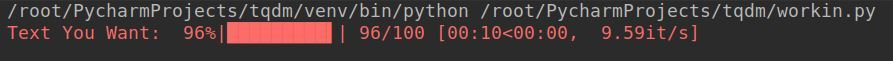• ascii: You can use ASCII characters to fill the progress bar as per your liking.Syntax:

tqdm (self, iterable, ascii= “123456789\$”, desc=”Text You Want”)

Example:

 `from` `tqdm ``import` `tqdm` `from` `time ``import` `sleep` ` ` ` ` `for` `i ``in` `tqdm(``range``(``0``, ``100``), ` `              ``ascii ``=``"123456789\$"``):` `    ``sleep(.``1``)`

Output: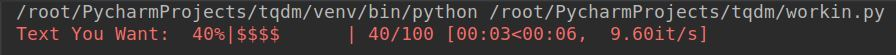• unit: The default unit of time is “it” and can be changed by using this parameter to your preferred unit.Syntax:
`tqdm (self, iterable, unit= “ ticks”)`

Example:

 `from` `tqdm ``import` `tqdm` `from` `time ``import` `sleep` ` ` ` ` `for` `i ``in` `tqdm(``range``(``0``, ``100``), unit ``=``" ticks"``, ` `              ``desc ``=``"Text You Want"``):` `    ``sleep(.``1``)`

Output: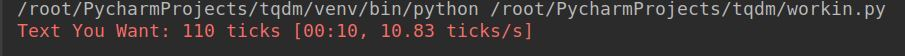• initial
The initial value of the progress bar starts from 0. If you wish to change this, you can use this parameter to initialize the progress bar from the value you wish
Syntax:
`tqdm (self, iterable, initial=50)`

Example:

 `from` `tqdm ``import` `tqdm` `from` `time ``import` `sleep` ` ` ` ` `for` `i ``in` `tqdm(``range``(``0``, ``100``), initial ``=` `50``, ` `              ``desc ``=``"Text You Want"``):` `    ``sleep(.``1``)`

Output: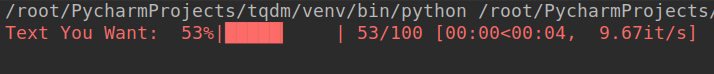The counter would start from 50 and the progress bar would disappear after the final counter is reached. The loop would still run until the iteration is complete.

Last Updated on November 13, 2021 by admin

## How to install pip in macOS ?How to install pip in macOS ?

Before we start with how to install pip for Python on macOS, let’s first go

## Python statistics | mean() functionPython statistics | mean() function

Python statistics | mean() function Python is a very popular language when it comes to

## Python GeneratorsPython Generators

Generators in Python There are two terms involved when we discuss generators. Generator-Function : A generator-function

## Add multiple columns to dataframe in PandasAdd multiple columns to dataframe in Pandas

Add multiple columns to dataframe in Pandas In Pandas, we have the freedom to add

## Pandas DataFrame.fillna() to replace Null values in dataframePandas DataFrame.fillna() to replace Null values in dataframe

Pandas DataFrame.fillna() to replace Null values in dataframe Python is a great language for doing

## Python – Find most frequent element in a listPython – Find most frequent element in a list

Python | Find most frequent element in a list Given a list, find the most

## Find size of a list in PythonFind size of a list in Python

Find size of a list in Python In Python, List is a collection data-type which

## Create a Pandas DataFrame from List of DictsCreate a Pandas DataFrame from List of Dicts

Pandas DataFrame is a 2-dimensional labeled data structure with columns of potentially different types. It